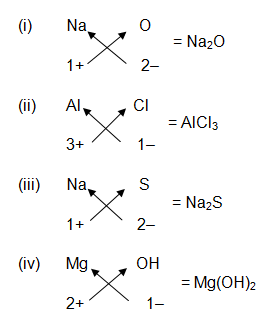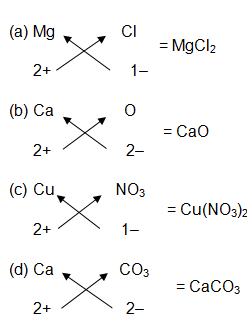# Formulae of Simple Compounds

The chemical formula of simple compound represents the composition of a molecule of the compound in terms of the symbol of these elements present in it. In other words, the formula of simple compound tells us the kind of atoms as well as the number of atoms of various elements present in one molecule of the compound.

In the formula of compounds, the elements are represented by their symbols and the number of atoms of each element are indicated by writing the digits 2, 3, 4 etc. as subscripts on the right hand side bottom of the symbol. But 1 atoms of any element is not represented specifically by writing as subscript. The symbol of element alone is enough to convey that.

Ionic compounds –

Ionic compounds are formed by the combination between metals and non-metals. While writing formula of ionic compounds, the metal element is written on the left hand side and the non-metal element is written on the right hand side. In naming the ionic compound, the metal element is named as such but the name of non-metal element is changed to have the ending ‘ide’ (like oxide, chloride, sulphide etc.).

A compound that is made up two elements is called a binary compound. All ionic compounds are binary compounds since they are made up of a metal and a non-metal.

Molecular compounds –

Molecular compounds are formed by the combination between two different non-metal elements. While writing the formula of a molecular compound, the less electronegative non-metal element is written on the left hand side whereas the more electronegative non-metal element is written on the right hand side. In naming the molecular compounds, the name of less electronegative non-metal is written as such but the name of more electronegative non-metal is changed to have the ending ‘ide’.

When there is more than one atom of an element in a molecular compound then the number of atoms is indicated by using appropriate prefixes in the formula. 1 atom is indicated by using the prefix ‘mono’; 2 atoms are indicated by using the prefix ‘di’; 3 atoms are indicated by using the prefix ‘tri’; 4 atoms are indicated by using the prefix ‘tetra’; and 5 atoms are indicated by using the prefix ‘penta’.

For example –

• CO is named as carbon monoxide(here the prefix ‘mono’ in monoxide means 1 oxygen atom)
• CO2 is named as carbon dioxide (here the prefix ‘di’ in dioxide means 2 oxygen atoms)
• PCl3 is named as phosphorous trichloride (here the prefix ‘tri’ in trichloride indicates 3 chlorine atoms)
• CCl4 is named as carbon tetrachloride (here the prefix ‘tetra’ in tetrachloride indicates 4 chlorine atoms)
• PCl5 is named as phosphorous pentachloride (here the prefix ‘penta’ in pentachloride indicates 5 chlorine atoms)

The prefixes are needed in naming binary molecular compounds in those cases where the same two non-metal elements form a number of different compounds having different number of atoms. For example, the same two non-metals nitrogen and oxygen can combine to form six different compounds such as nitrogen monoxide (NO), nitrogen dioxide (NO2), dinitrogen monoxide (N2O), dinitrogen trioxide (N2O3), dinitrogen tetroxide (N2O4) and dinitrogen pentoxide (N2O5). So, it is necessary to use prefixes in such case.

If, however, two non-metal elements form just one compound, then prefixes are not used in naming such compounds. For example, hydrogen and chlorine form just one compound HCl. So, HCl is named as hydrogen chloride and not as hydrogen monochloride.

If hydrogen is the first element in a formula, then no prefix like di, tri etc. are put before the name ‘hydrogen’, even if it has more than one hydrogen atoms. For example – the compound H2S is named as hydrogen sulphide and not as dihydrogen sulphide.

There are some molecular compounds that are known by their common names and not by their scientific names. For example – though the compound H2O is actually hydrogen monoxide but it is known by its common name water. Similarly, though the compound NH3 is actually nitrogen tri hydride but it is known by its common name which is ammonia.

### Questions based on formulae of simple compounds from NCERT textbook –

Page 39

Question 1:-

Write down the chemical formula of –

• Sodium oxide
• Aluminium chloride
• Sodium sulphide
• Magnesium hydroxide

Answer :-Question 2:-

Write down the names of compounds represented by the following formulae:

• Al2(SO4)3
• CaCl2
• K2SO4
• KNO3
• CaCO3

Answer 2:-

• Aluminium sulphate
• Calcium chloride
• Potassium sulphate
• Potassium nitrate
• Calcium carbonate

Question 3:-

What is meant by the term chemical formula?

Answer 3:-

The chemical formula of a compound represents the composition of a molecule of the compound in terms of the symbols of the elements present in it. For example, the chemical formula of water is H2O tells us that one molecule of water is made up of 2 hydrogen atoms and 1 oxygen atom chemically combined together.

Pages 43 and 44

Question 4:-

Write the chemical formulae of the following :

• Magnesium chloride
• Calcium oxide
• Copper nitrate
• Aluminium chloride
• Calcium carbonate

Answer :-Question 5:-

Give names of the elements present in the following compounds:

• Quick lime
• Hydrogen bromide
• Baking soda
• Potassium sulphate

Answer:-

• Quick lime is calcium oxide, CaO. The elements present in quick lime are: calcium (Ca) and oxygen (O).
• Hydrogen bromide is HBr. It contains: hydrogen (H) and bromine (Br).
• Baking soda is sodium Hydrogen carbonate, NaHCO3. The elements present in baking soda are: sodium (Na), hydrogen (H), carbon (C) and oxygen (O).
• Potassium sulphate is K2SO4. The elements present in potassium sulphate are: potassium (K), sulphur (S), oxygen (O).

Conclusion – The formula of simple compounds is used to convey the information about the elements present in the compound and their quantity. The ionic compounds are made up of metals and non-metals and during their naming the metal is named first and then the non-metal. In molecular compounds, the formula is written with prefixes such as mono, di, tri etc. to indicate the number of the element present however no prefix is applied before hydrogen.